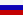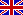­ˇ˝eng

## Scope and Topics

The journal publishes articles on the following scientific specialties and branches of science:

• 1.1.4 - Probability theory and mathematical statistics,
• 1.1.5 - Mathematical logic, algebra, number theory and discrete mathematics,
• 1.1.6 - Computational mathematics,
• 1.2.1 - Artificial intelligence and machine learning,
• 1.2.2 - Mathematical modeling, numerical methods and program systems,
• 1.2.3 - Theoretical computer science, cybernetics
• 2.3.1 - Systems analysis, management and information processing,
• 2.3.4 - Management in social and economic systems